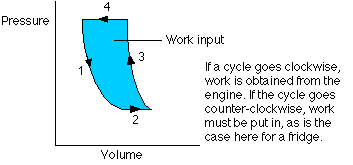Heat engines and the second law

12-10-99

Sections 15.5 - 15.6

The second law of thermodynamics

The second law of thermodynamics comes in more than one form, but let's state in a way that makes it obviously true, based on what you've observed from simply being alive.

The second law states that heat flows naturally from regions of higher temperature to regions of lower temperature, but that it will not flow naturally the other way.

Heat can be made to flow from a colder region to a hotter region, which is exactly what happens in an air conditioner, but heat only does this when it is forced. On the other hand, heat flows from hot to cold spontaneously.

Heat engines

We'll move on to look at heat engines, which are devices that use heat to do work. A basic heat engine consists of a gas confined by a piston in a cylinder. If the gas is heated, it expands, moving the piston. This wouldn't be a particularly practical engine, though, because once the gas reaches equilibrium the motion would stop. A practical engine goes through cycles; the piston has to move back and forth. Once the gas is heated, moving the piston up, it can be cooled and the piston will move back down. A cycle of heating and cooling will move the piston up and down.

A necessary component of a heat engine, then, is that two temperatures are involved. At one stage the system is heated, at another it is cooled.

In a full cycle of a heat engine, three things happen:

1. Heat is added. This is at a relatively high temperature, so the heat can be called QH.
2. Some of the energy from that input heat is used to perform work (W).
3. The rest of the heat is removed at a relatively cold temperature (QC).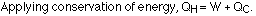The following diagram is a representation of a heat engine, showing the energy flow: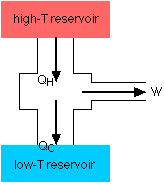An important measure of a heat engine is its efficiency: how much of the input energy ends up doing useful work? The efficiency is calculated as a fraction (although it is often stated as a percentage):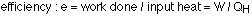Work is just the input heat minus the rejected heat, so: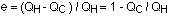Note that this is the maximum possible efficiency for an engine. In reality there will be other losses (to friction, for example) that will reduce the efficiency.

Carnot's principle

How can an engine achieve its maximum efficiency? It must operate using reversible processes: a reversible process is one in which the system and the surroundings can be returned to state they were in before the process began. If energy is lost to friction during a process, the process is irreversible; if energy is lost as heat flows from a hot region to a cooler region, the process is irreversible. The efficiency of an engine using irreversible processes can not be greater than the efficiency of an engine using reversible processes that is working between the same temperatures. This is known as Carnot's principle, named after Sadi Carnot, a French engineer.

For any reversible engine (known as a Carnot engine) operating between two temperatures, TH and TC, the efficiency is given by:The efficiency is maximized when the cold reservoir is as cold as possible, and the hot temperature is as hot as possible.

The third law

The third law of thermodynamics states this : it is impossible to reach absolute zero. This implies that a perpetual motion machine is impossible, because the efficiency will always be less than 1.

Refrigerators, air conditioners, etc.

A device such as a refrigerator or air conditioner, designed to remove heat from a cold region and transfer it to a hot region, is essentially a heat engine operating in reverse, as the following energy flow diagram shows: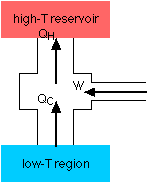A refrigerator, consisting of a fluid pumped through a closed system, involves a four-step process. An air conditioner works the same way.

• Step 1 - The fluid passes through a nozzle and expands into a low-pressure area. Similar to the way carbon dioxide comes out of a fire extinguisher and cools down, the fluid turns into a gas and cools down. This is essentially an adiabatic expansion.
• Step 2 - The cool gas is in thermal contact with the inner compartment of the fridge; it heats up as heat is transferred to it from the fridge. This takes place at constant pressure, so it's an isobaric expansion.
• Step 3 - The gas is transferred to a compressor, which does most of the work in this process. The gas is compressed adiabatically, heating it and turning it back to a liquid.
• Step 4 - The hot liquid passes through coils on the outside of the fridge, and heat is transferred to the room. This is an isobaric compression process.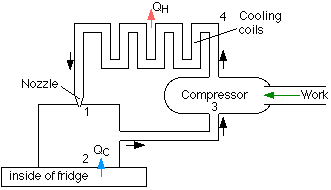A refrigerator is rated by something known as the coefficient of performance, which is the ratio of the heat removed from the fridge to the work required to remove it: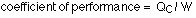The P-V graph for a refrigerator cycle

The P-V (pressure-volume) graph is very useful for calculating the work done. For any kind of heat engine or refrigerator (reverse heat engine), the processes involved form a cycle on the P-V graph. The work is the area of the enclosed region on the graph. The diagram for a refrigerator is a little more complicated than this because of the two phase changes involved, but this is basically what it looks like: